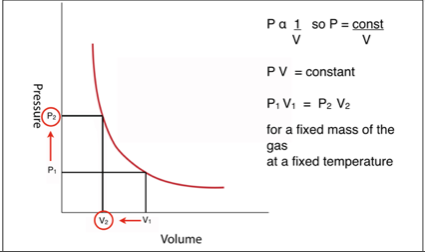### Boyle’s Law

Boyle’s Law is that: for a fixed mass of gas at a constant temperature the pressure is inversely proportional to the volume.

The Law was formulated empirically, that is, when it was written it fitted in with observations and experiment.  The kinetic theory of gases offers an explanation.

The molecules of a gas move around constantly. As they hit the sides of the container the change in momentum exerts a force on the container which is what causes the pressure of the gas.

If the temperature remains the same the molecules move with the same average speed.

However, the container is made smaller, they hit the sides more often and therefore the pressure is higher. For example if the volume was halved they would hit the sides twice as often so the pressure would be doubled.

A graph of pressure plotted against volume (with the temperature remaining constant) would look like this.Pressure plotted against volume for gas at constant temperature

The law is often written in the form of a “before and after” equation. That is the pressure multiplied by the volume before the change equals the pressure multiplied by the volume after.

You can download a PDF copy of these notes here:    Boyle's Law notes

Other pages on the Kinetic theory of gases and the gas Laws are: